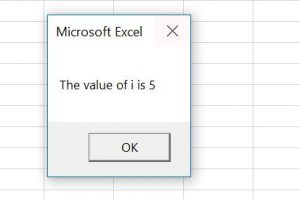# VBA End

In VBA, to end ALL code execution use the End command.

``End``

When the execution of the code comes to End, it will immediately finish the execution and leave the program.

If you want to learn how to exit a Sub on Error, click on this link: VBA On Error Exit Sub

If you want to learn how to exit a Sub or Function, click on this link: VBA Exit Sub or Function

## Exit all Code in VBA

You will see on the example what happens when we use the End command in VBA. We created a Sub EndSub, which has the End command inside. This Sub is called from CallEndSub. Here is the code:

``````Private Sub EndSub()

Dim i As Integer

For i = 1 To 10
If i = 5 Then
MsgBox "The value of i is " & i
End
End If
Next i

End Sub

Private Sub CallEndSub()
Call EndSub
MsgBox "End Sub"
End Sub``````

In the EndSub, we first enter the For Loop if the value of i is less than 10:

``````For i = 1 To 10

Next i``````

After that we check if the value of i is equal to 5, using the If command. If the value is 5, we return the message box with the value of i and use the command End.

``````If i = 5 Then

MsgBox "The value of i is " & i

End

End If``````

If the condition is not met, the following statement increases i by 1 and enters in the For loop again:

``Next i``

In the CallEndSub, we first call the Sub EndSub:

``Call EndSub``

After that we return the Message box:

``MsgBox "End Sub"``

If you run the CallEndSub, it will first call the EndSub. If you execute this code in the debug mode, you will see that it will go through the loop 5 times. In the 5th iteration, the value of the variable i becomes 5 and the code enters in the If body. Now the MsgBox “The value of i is ” & i is executed and End after that:Image 1. VBA End

As you can see, the code execution is stopped right after the End command, so the MsgBox “End Sub” is never executed.

Stop searching for VBA code online. Learn more about AutoMacro - A VBA Code Builder that allows beginners to code procedures from scratch with minimal coding knowledge and with many time-saving features for all users!